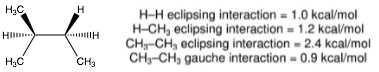# Problem: What is the total strain energy (in kcal/mol) of the least stable (highest energy) conformation possible for 2-methylbutane? All of the choices below are expressed in units of kcal/mol. A. 0          B. 0.9          C. 1.8           D. 2.7         E. 3.4F. 4.4        G. 4.6         H. 5.8           I. 7.2           J. None of these

###### FREE Expert Solution
91% (499 ratings)
###### FREE Expert Solution

We’re being asked to calculate the total strain energy (in kcal/mol) for the least stable conformation of 2-methylbutane.

Recall that strain energy is the energy a compound needs to overcome to change from one conformation to the other. It is the sum of the steric interactions present in a Newman projection.

For this problem, we need to do the following steps:

Step 1: Draw the Newman projection for the given structure.

Step 2: Identify the steric interactions and calculate the total strain energy.

91% (499 ratings)###### Problem Details

What is the total strain energy (in kcal/mol) of the least stable (highest energy) conformation possible for 2-methylbutane? All of the choices below are expressed in units of kcal/mol.

A. 0          B. 0.9          C. 1.8           D. 2.7         E. 3.4

F. 4.4        G. 4.6         H. 5.8           I. 7.2           J. None of theseWhat scientific concept do you need to know in order to solve this problem?

Our tutors have indicated that to solve this problem you will need to apply the Barrier To Rotation concept. You can view video lessons to learn Barrier To Rotation. Or if you need more Barrier To Rotation practice, you can also practice Barrier To Rotation practice problems.

What is the difficulty of this problem?

Our tutors rated the difficulty ofWhat is the total strain energy (in kcal/mol) of the least s...as medium difficulty.

How long does this problem take to solve?

Our expert Organic tutor, Jonathan took 5 minutes and 28 seconds to solve this problem. You can follow their steps in the video explanation above.

What professor is this problem relevant for?

Based on our data, we think this problem is relevant for Professor Arrey's class at Capital University.